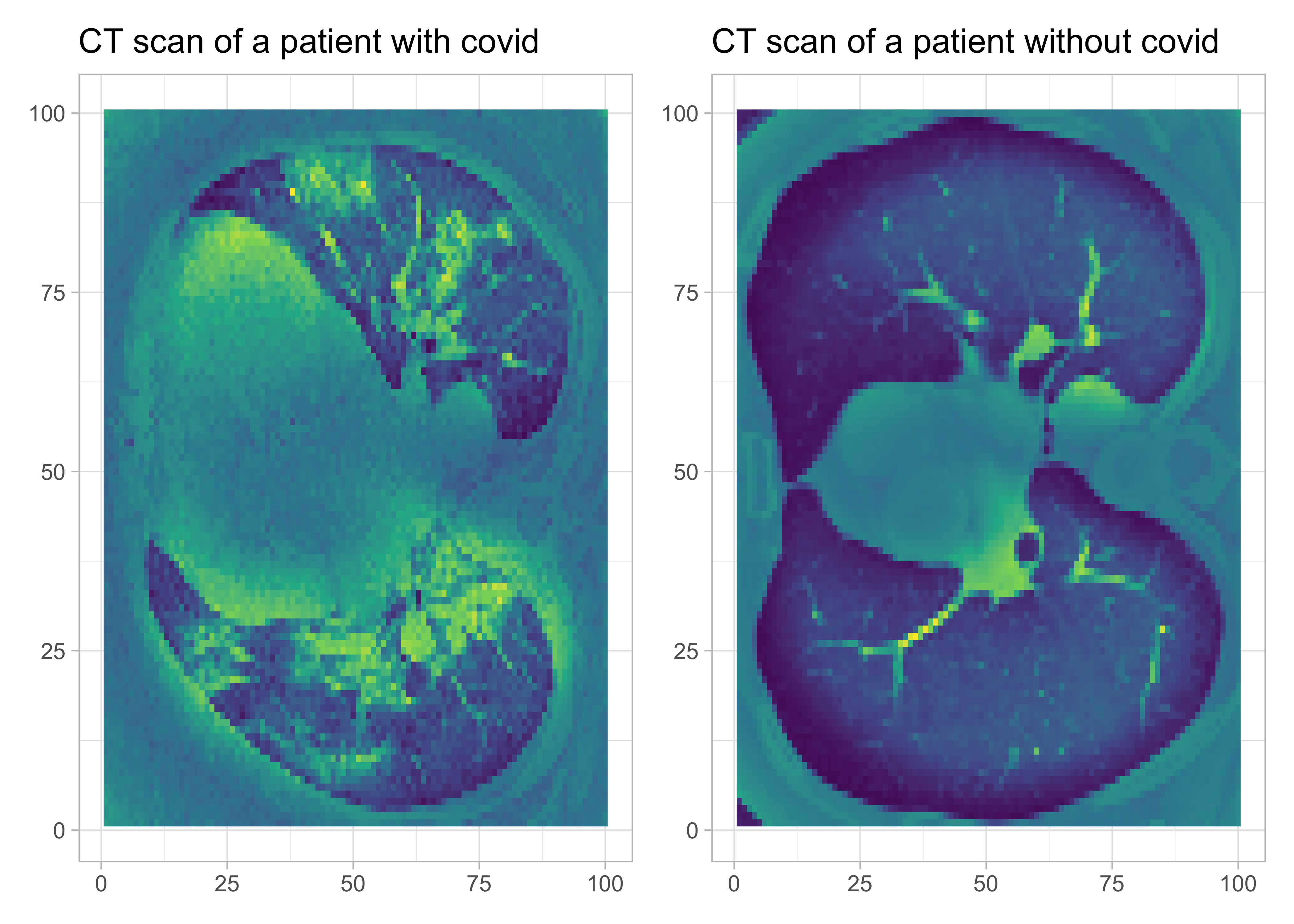Want to share your content on R-bloggers? click here if you have a blog, or here if you don't.

Here I illustrate how to train a CNN with Keras in R to predict from patients’ CT scans those who will develop severe illness from Covid.

# Motivation

Michael Blum tweeted about the STOIC2021 – COVID-19 AI challenge. The main goal of this challenge is to predict from the patients’ CT scans who will develop severe disease.

Given my recent interest in machine learning, this challenge peaked my interest. Although `Python` is the machine learning lingua franca, it is possible to train a convolutional neural network (CNN) in `R` and perform (binary) image classification.

Here, I will use an `R` interface to `Keras` that allows training neural networks. The other thing is that the dataset shared for the challenge is big, like 280Go big. It took me a day to download it. For the sake of illustration, I will use a similar but much lighter dataset from a Kaggle repository https://www.kaggle.com/plameneduardo/sarscov2-ctscan-dataset.

The code is available on GitHub as usual https://github.com/oliviergimenez/bin-image-classif.

First things first, load the packages we will need.

```library(tidyverse)
theme_set(theme_light())
library(keras)
```

# Read in and process data

We will need a function to process images, I’m stealing that one written by Spencer Palladino.

```process_pix <- function(lsf) {
img <- lapply(lsf, image_load, grayscale = TRUE) # grayscale the image
arr <- lapply(img, image_to_array) # turns it into an array
arr_resized <- lapply(arr, image_array_resize,
height = 100,
width = 100) # resize
arr_normalized <- normalize(arr_resized, axis = 1) #normalize to make small numbers
return(arr_normalized)
}
```

Process pix for patients with Covid, and reshape. Idem for pix for patients without Covid.

```# with covid
lsf <- list.files("dat/COVID/", full.names = TRUE)
covid <- process_pix(lsf)
covid <- covid[,,,1] # get rid of last dim
covid_reshaped <- array_reshape(covid, c(nrow(covid), 100*100))
# without covid
lsf <- list.files("dat/non-COVID/", full.names = TRUE)
ncovid <- process_pix(lsf)
ncovid <- ncovid[,,,1] # get rid of last dim
ncovid_reshaped <- array_reshape(ncovid, c(nrow(ncovid), 100*100))
```

We have 1252 CT scans of patients with Covid, and 1229 without.

Let’s visualise these scans. Let’s pick a patient with Covid, and another one without.

```scancovid <- reshape2::melt(covid[10,,])
plotcovid <- scancovid %>%
ggplot() +
aes(x = Var1, y = Var2, fill = value) +
geom_raster() +
labs(x = NULL, y = NULL, title = "CT scan of a patient with covid") +
scale_fill_viridis_c() +
theme(legend.position = "none")
scanncovid <- reshape2::melt(ncovid[10,,])
plotncovid <- scanncovid %>%
ggplot() +
aes(x = Var1, y = Var2, fill = value) +
geom_raster() +
labs(x = NULL, y = NULL, title = "CT scan of a patient without covid") +
scale_fill_viridis_c() +
theme(legend.position = "none")
library(patchwork)
plotcovid + plotncovid
```Put altogether and shuffle.

```df <- rbind(cbind(covid_reshaped, 1), # 1 = covid
cbind(ncovid_reshaped, 0)) # 0 = no covid
set.seed(1234)
shuffle <- sample(nrow(df), replace = F)
df <- df[shuffle, ]
```

Sounds great. We have everything we need to start training our convolutional neural network model.

# Convolutional neural network (CNN)

Build our training and testing datasets using a 80/20 split.

```set.seed(2022)
split <- sample(2, nrow(df), replace = T, prob = c(0.8, 0.2))
train <- df[split == 1,]
test <- df[split == 2,]
train_target <- df[split == 1, 10001] # label in training dataset
test_target <- df[split == 2, 10001] # label in testing dataset
```

Build our model. I use a single layer, but it’s easy to pipe others on top of it.

```model <- keras_model_sequential() %>%
layer_dense(units = 512, activation = "relu") %>%
layer_dropout(0.4) %>%
layer_dense(units = 256, activation = "relu") %>%
layer_dropout(0.3) %>%
layer_dense(units = 128, activation = "relu") %>%
layer_dropout(0.2) %>%
layer_dense(units = 2, activation = 'softmax')
```

Compile it.

```model %>%
loss = 'binary_crossentropy',
metrics = c('accuracy'))
```

We use one-hot encoding with function `to_categorical()`, aka dumming coding in statistics.

```train_label <- to_categorical(train_target)
test_label <- to_categorical(test_target)
```

Now fit model.

```fit_covid <- model %>%
fit(x = train,
y = train_label,
epochs = 25,
batch_size = 512, # try also 256, 512
verbose = 2,
validation_split = 0.2)
```

Visualize performances. Not too bad. No over/under-fitting. Accuracy and loss are fine.

```plot(fit_covid)
```Performance on test dataset?

```model %>%
evaluate(test, test_label)

## loss accuracy
## 0.2800467 0.8612245
```

Let’s do some predictions on the test data, and compare with ground truth.

```predictedclasses <- model %>%
predict_classes(test)
table(Prediction = predictedclasses,
Actual = test_target)

## Actual
## Prediction 0 1
## 0 176 0
## 1 68 246
```

Pretty cool. Only a few patients are misclassified. Let’s save our model for further use.

```save_model_tf(model, "model/covidmodel") # save the model
```

I’m happy with the results. In general however, we need to find ways to improve the performances. A few tips here with examples implemented in `Keras` with `R` there.